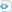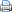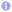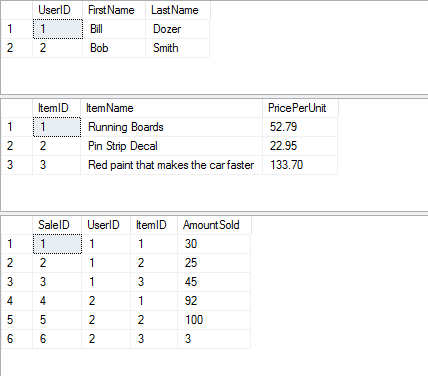# MSSQL Sub Query

This is written using Microsoft SQL Server however a lot of this can carry over just fine to other SQL variants with little change. I am also using SQL Management Studio 2016 when writing this query. This can be found here.

Sub queries come handy when you need to return data from multiple tables but need that data Summed up or some form of calculation done on them. This isn’t the only scenario where these are useful but in this example that’s what I will be doing.

The example below does not require you to create any tables as everything is done in memory.

Creating our tables:

 Source code```DECLARE @Users TABLE (
UserID INT IDENTITY(1,1) PRIMARY KEY,
FirstName VARCHAR(25),
LastName VARCHAR(25)

);

DECLARE @items TABLE (
ItemID INT IDENTITY(1,1) PRIMARY KEY,
ItemName VARCHAR(150),
PricePerUnit NUMERIC(5,2)

);

DECLARE @sales TABLE (
SaleID INT IDENTITY(1,1) PRIMARY KEY,
UserID INT,
ItemID INT,
AmountSold INT

);```

The “@” tells SQL Server that we are creating a variable and in this case the data type for that variable is a table.

Notes:
IDENTITY(1,1) means that this field auto increments. This is great for Primary keys so you do not have to know what the last ID was, it will do that for you and insert it.

Now we want to insert data into these table so we have something to lookup later on.

Insert statements:

 Source code```INSERT INTO @Users VALUES('Bill','Dozer');
INSERT INTO @Users VALUES('Bob','Smith');

INSERT INTO @items VALUES('Running Boards',52.79);
INSERT INTO @items VALUES('Pin Strip Decal',22.95);
INSERT INTO @items VALUES('Red paint that makes the car faster',133.70);

INSERT INTO @sales VALUES(1,1,30);
INSERT INTO @sales VALUES(1,2,25);
INSERT INTO @sales VALUES(1,3,45);
INSERT INTO @sales VALUES(2,1,92);
INSERT INTO @sales VALUES(2,2,100);
INSERT INTO @sales VALUES(2,3,3);```

Nothing much to say here other than to make sure your data follows the constraints you set when you created the fields in the tables.
If you wish to see the data that was put in you can use these SELECT statements:

You will see this:Now I want to know how many items each User sold and how much money they made each for those sales as totals.

So this is the data we want. We want the user’s name as well as the Total Stock sold (this would be a sum of the items they sold) and a total dollar amount that was sold per person.

So our resulting table should resemble something like this:

Now that we know what we want we now need to build a query that gets us this information.

First off we need the user name:

The above gives us every user in the Users table and displays their name like Smith, Bob.
We also gave the @Users table an Alias of usr. This becomes important in future steps since we will need to reference this again in our sub queries.

Now we want to get the Total Stock Sold per user. So we are going to add the following to the query we already started:

 Source code```(
SELECT
SUM(AmountSold)
FROM
@Sales AS Sa
WHERE
Sa.UserID = usr.UserID
) AS 'Total Stock Sold'```

Sub queries are always encased in parentheses. So this query uses the SUM function on AmountSold From the @Sales table. We also give this table an Alias. SUM will take every row in that column (AmountSold) and add them all together. However since we want to only sum the AmountSold and have it displayed for the correct user we have to tell it to only sum it up for the current User we are on. So we add in a WHERE clause and tell it to do it on records that match. So in this case we want it to only SUM where Sa(@Sales table) UserID matches our usr(Users table) UserID. This is why I said the Alias prior was important since we have to use it to do the matching.

Now that this is done we want to now get the Sales Total. We will now add the following to there query we have been working on:

 Source code```(
SELECT
SUM(PricePerUnit * Sa.AmountSold)
FROM
@items AS It
JOIN
@sales Sa  ON Sa.ItemID = It.ItemID
WHERE
usr.UserID = Sa.UserID
) AS 'Sales Total'```

This query works much like the other however now we are doing some multiplication in the SUM function. We multiply those 2 fields together and them sum all those results together to get the total Sales for that user.

The key difference in this one is that we are also now a JOIN. JOINs are important when you are trying to select from more than one table. In this case we want to match records from the @Sales table and the @Items table. The reason we do this is because we need the PricePerUnit in order to do our calculations.

So now that this is all done the final statement will look like this:

 Source code```SELECT
LastName + ', ' + FirstName AS 'Name',
(
SELECT
SUM(AmountSold)
FROM
@Sales AS Sa
WHERE
Sa.UserID = usr.UserID
) AS 'Total Stock Sold',
(
SELECT
SUM(PricePerUnit * Sa.AmountSold)
FROM
@items AS It
JOIN
@sales Sa  ON Sa.ItemID = It.ItemID
WHERE
usr.UserID = Sa.UserID
) AS 'Sales Total'
FROM @Users AS usr```This entry was posted in Databases, MSSQL. Bookmark the permalink.